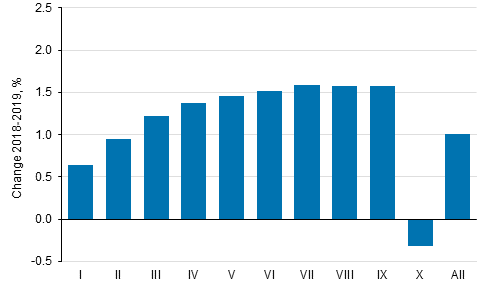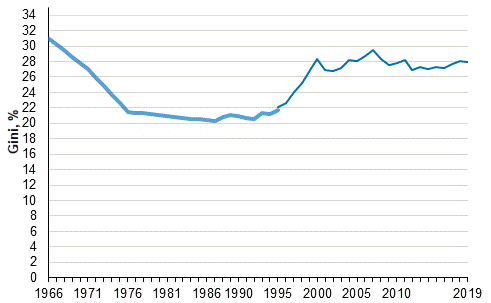Data published after 5 April 2022 can be found on the renewed website.

Go to the new statistics page

Published: 16 December 2020

# Income differentials decreased slightly in 2019

Statistics Finland's income distribution statistics show that income differentials decreased slightly in 2019 compared with the previous year. Real income increased in all income deciles except in the highest-income decile. The income level of the population's highest-income decile decreased by 0.3 per cent in real terms from 2018 to 2019. The income of medium income earners (income deciles V to IX) increased by 1.5 per cent and the income of the four lowest income deciles by 1.1 per cent. The income level of the lowest-income decile grew by 0.6 per cent.

Change in average real income (%) by income decile from 2018 to 2019Income decile: the population is arranged according to income and divided into ten equal parts, each of which has around half a million persons. The first (I) income decile is the lowest-income decile and the tenth (X) is the highest-income decile. Income concept: equivalent disposable monetary income (incl. realised capital gains) of household dwelling-unit, average per person. Equivalent income: the household-dwelling unit’s income divided by its consumption units (modified OECD-scale).

In 2019, the value of Gini coefficient describing relative income differentials was 27.9 which was 0.2 percentage points lower than in the previous year and on level with 2010. Compared with 1995, the Gini coefficient has grown by around 5.7 percentage points. Most of this is caused by the fast growth of income differentials at the end of the 1990s. The income differentials have been at their lowest in the mid-1980s. The Gini coefficient gets the value 0 if everyone receives the same amount of income and 100 if one income earner receives all the income. The higher the Gini coefficient, the bigger the relative income differentials are.

Development of income inequality 1966–2019, Gini coefficient (%)Persons belonging to the household-dwelling population, the household-dwelling unit's equivalent disposable monetary income (incl. realised capital gains). Sources: 1995 to 2019 Income distribution statistics’ total data; 1986 to 1995: Income distribution statistics’ sample data; 1966,1971,1976,1981 Household Budget Survey. The missing intervening years (1967 to 1985) have been linearly interpolated.

Source: Income distribution statistics 2019, Statistics Finland

Inquiries: Usva Topo 029 551 3036, Pekka Ruotsalainen 029 551 2610, Kaisa-Mari Okkonen 029 551 3408, toimeentulo.tilastokeskus@stat.fi

Head of Department in charge: Hannele Orjala

Publication in pdf-format (161.6 kB)

Tables

Tables in databases

Pick the data you need into tables, view the data as graphs, or download the data for your use.

Updated 16.12.2020

Referencing instructions:

Official Statistics of Finland (OSF): Income distribution statistics [e-publication].
ISSN=1799-1331. Income inequality (international comparison) 2019. Helsinki: Statistics Finland [referred: 4.6.2023].
Access method: http://www.stat.fi/til/tjt/2019/01/tjt_2019_01_2020-12-16_tie_001_en.html